# To write: the name of the postulate, that justifies the given statement. Given information:In /_AVB and /_NVK, /_A=/_N=60^(circ). The given figure is as follows: 12210203041.jpgQuestion
SimilarityTo write: the name of the postulate, that justifies the given statement.
Given information:In $$\displaystyle\triangle{A}{V}{B}{\quad\text{and}\quad}\triangle{N}{V}{K},\angle{A}=\angle{N}={60}^{{\circ}}$$.
The given figure is as follows: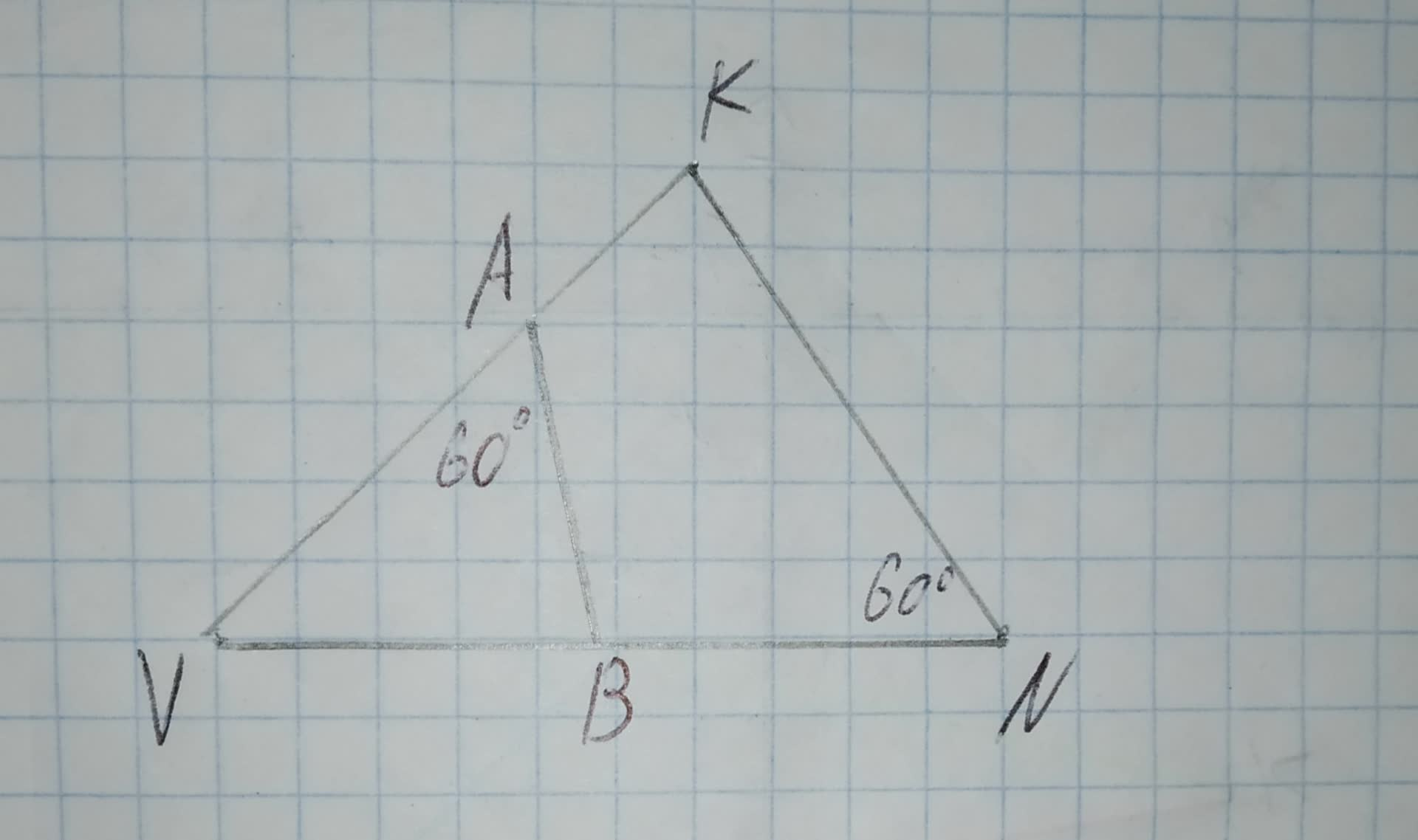2021-03-06
Angle-Angle similarity criterion states that if two angles of one triangle are equal to two angles of another triangle, the triangles are similar.
In $$\displaystyle\triangle{A}{V}{B}{\quad\text{and}\quad}\triangle{N}{V}{K}$$,
$$\displaystyle\angle{A}=\angle{N}{\quad\text{and}\quad}\angle{V}$$ is common angle
So, $$\displaystyle\triangle{A}{V}{B}\sim\triangle{N}{V}{K}$$ by Angle-Angle similarity theorem.
Hence,the theorem that justifies the given statement is Angle-Angle similarity theorem.

### Relevant QuestionsTo determine:Whether each pair of figures is similar and also write similarity statement and scale factor.
Given information:
Given the figure.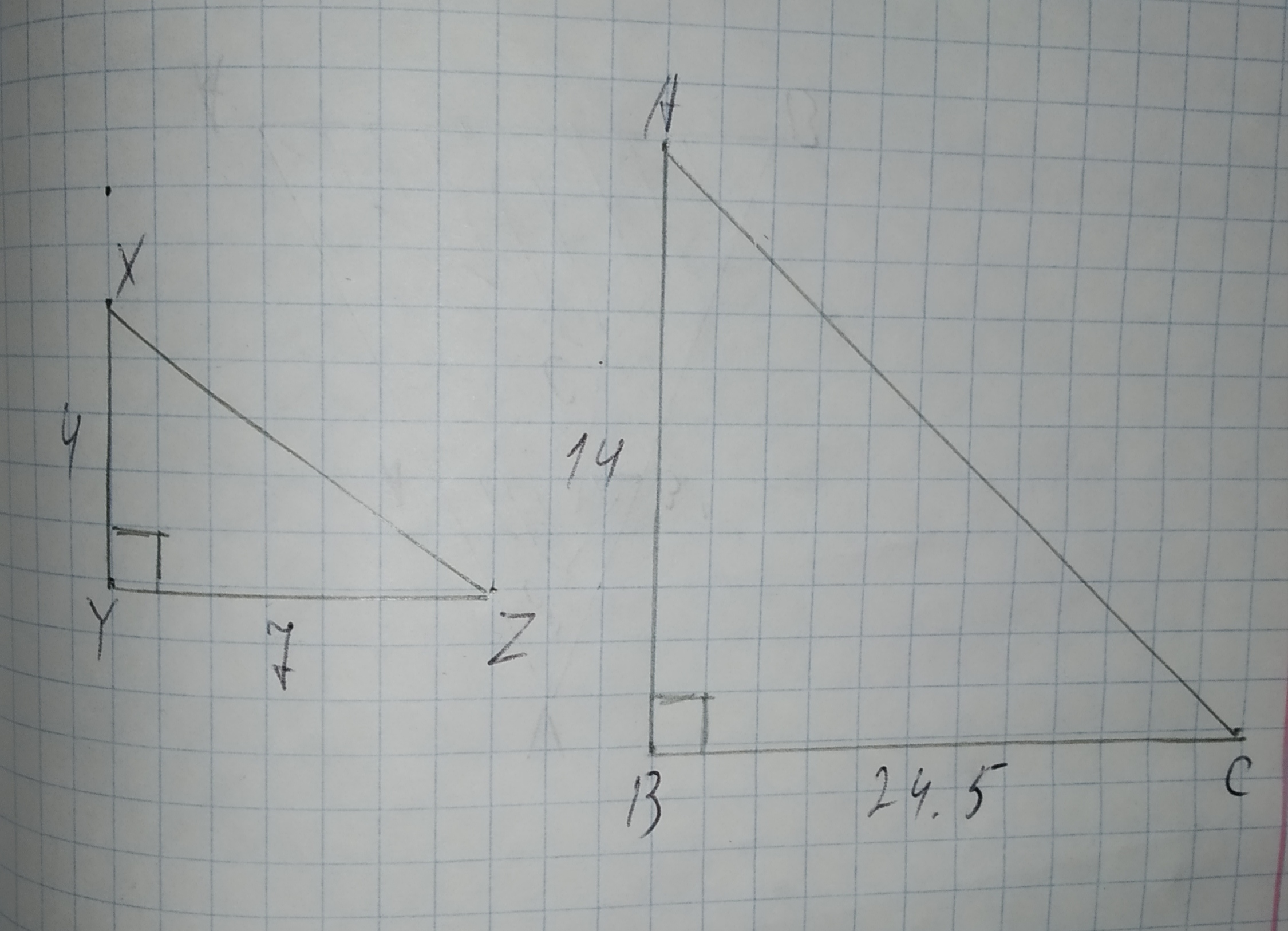Determine whether the triangles are similar.
If so, write a similarity statement and name the postulate or theorem you used. If not, explain.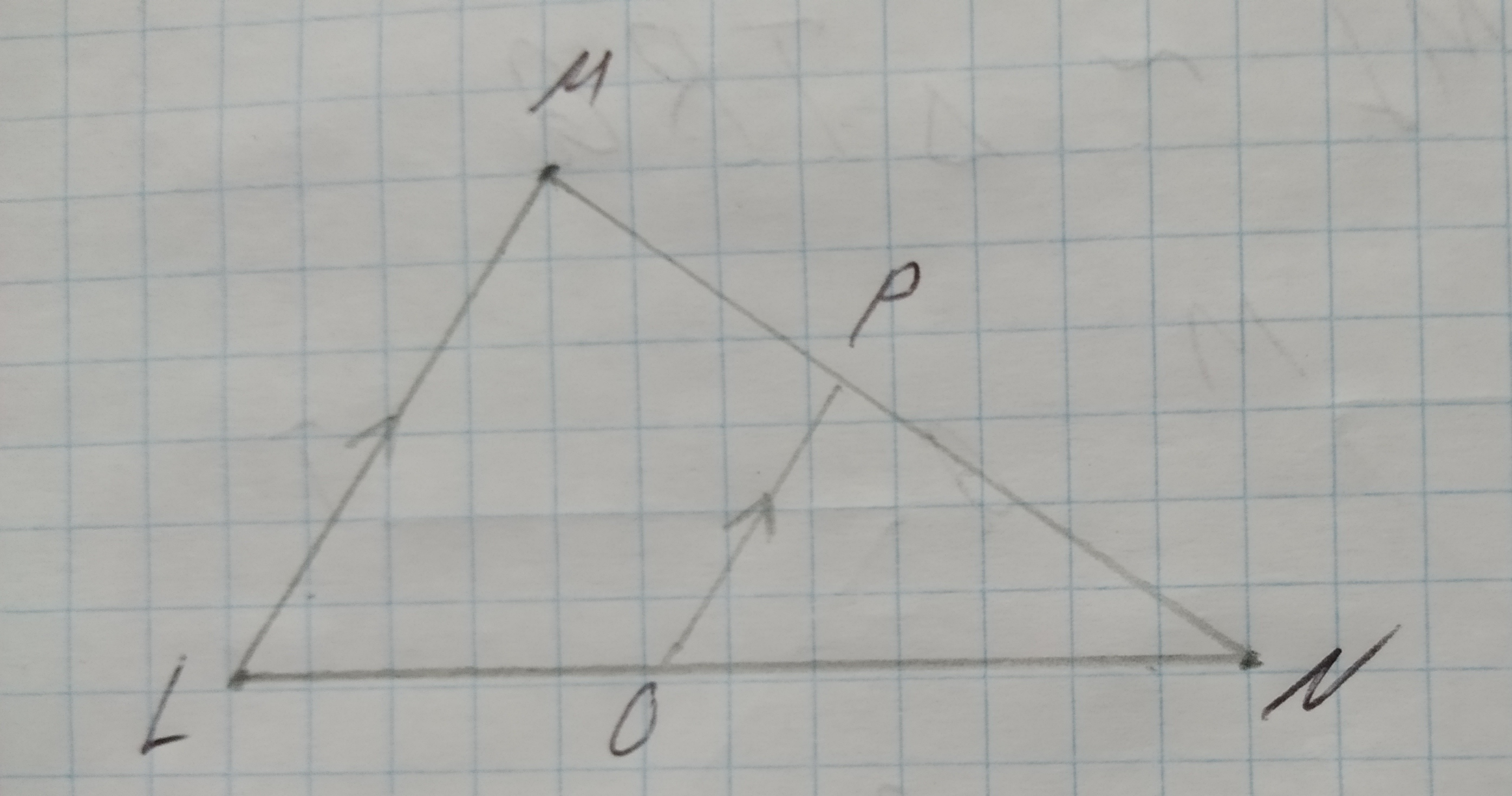To Complete:the statement WZ=? and RS=? in the figure shown.
Given:
Figure is shown below.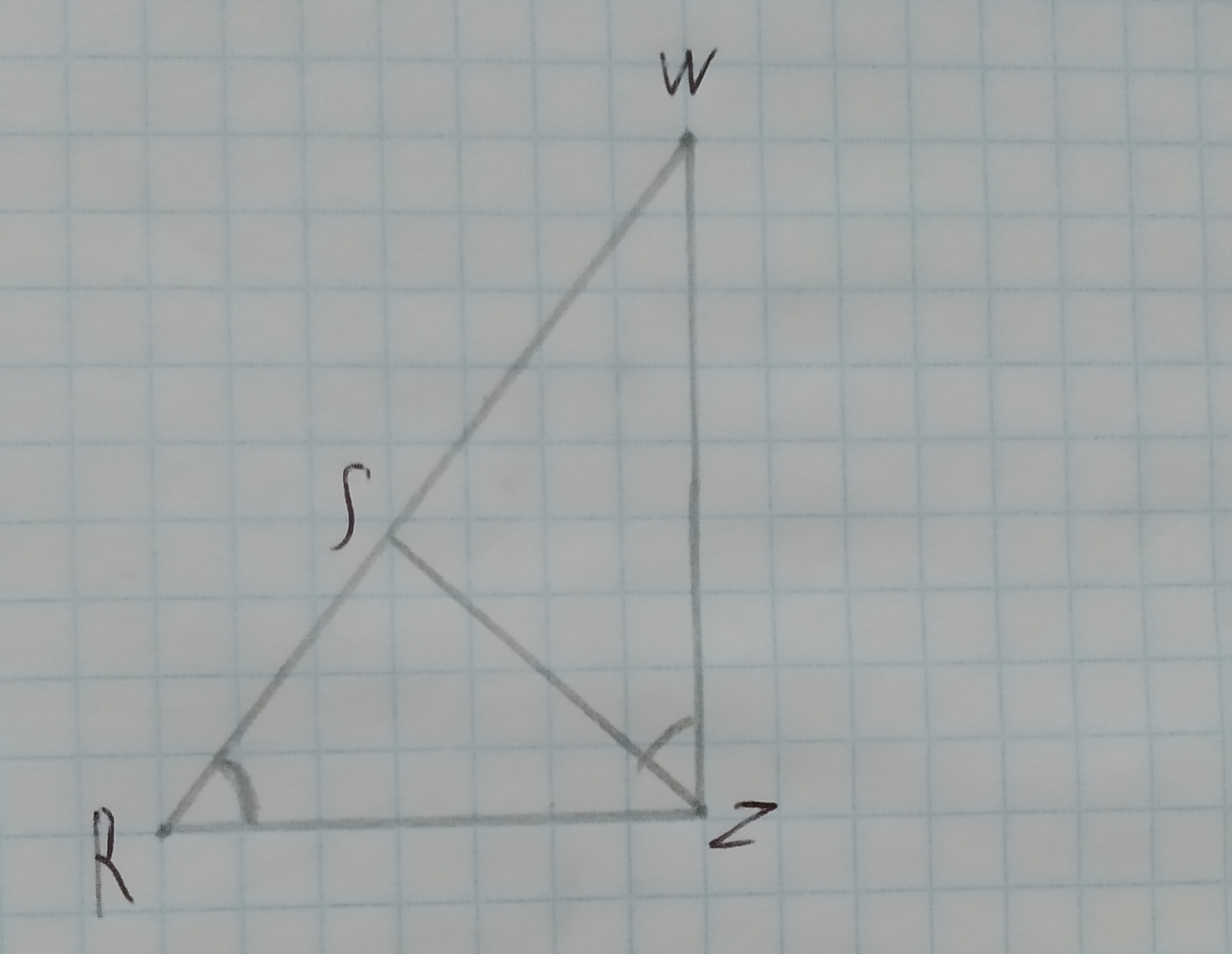RW=15, ZR=10 and ZS=8To Complete: the statement $$\displaystyle\frac{{{R}{W}}}{?}=\frac{{{Z}{R}}}{?}=\frac{{{W}{Z}}}{?}$$ in the figure shown,
Given:
Figure is shown below.To Complete: the statement $$\displaystyle\triangle{R}{W}{Z}=$$? in the figure shown.
Given:
Figure is shown below.To determine:To Find:the measure of side BB' in the figure shown such that VA' = 15, A'A=20 and A'B'=24
Given:
Figure is shown below.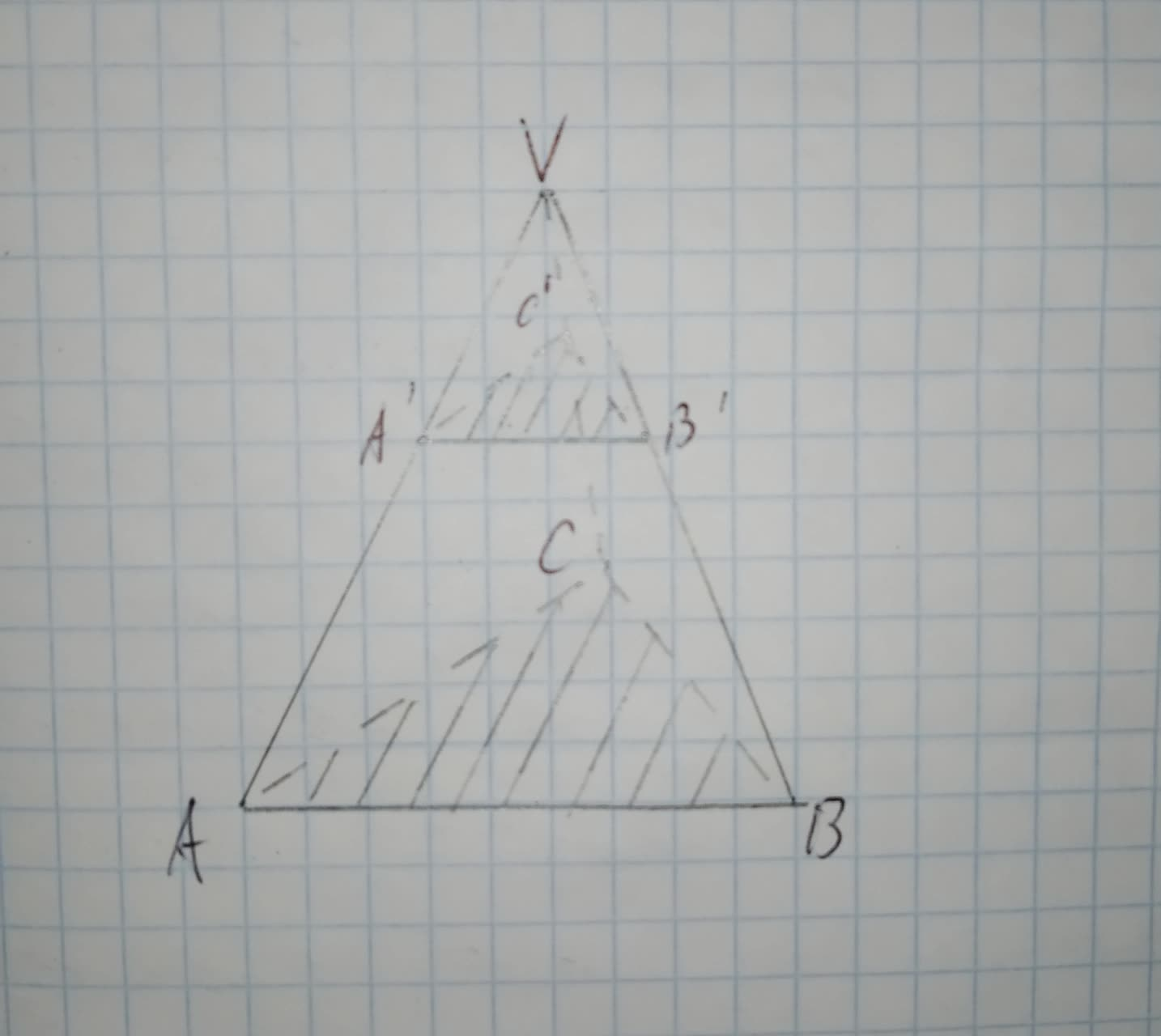To determine:To Find:the measure of side BB' in the figure shown such that VA' = 15, A'A=20 and VB=49
Given:
Figure is shown below.To determine:To Find:the measure of side VC in the figure shown such that VA' = 15, A'A=20 and VC'=18
Given:
Figure is shown below.Given $$\displaystyle\triangle{D}{E}{F}{\quad\text{and}\quad}\triangle{E}{G}{F}$$ in the diagram below, determine if the triangles are similar. If so, write a similarity statement, and state the criterion used to support your claim.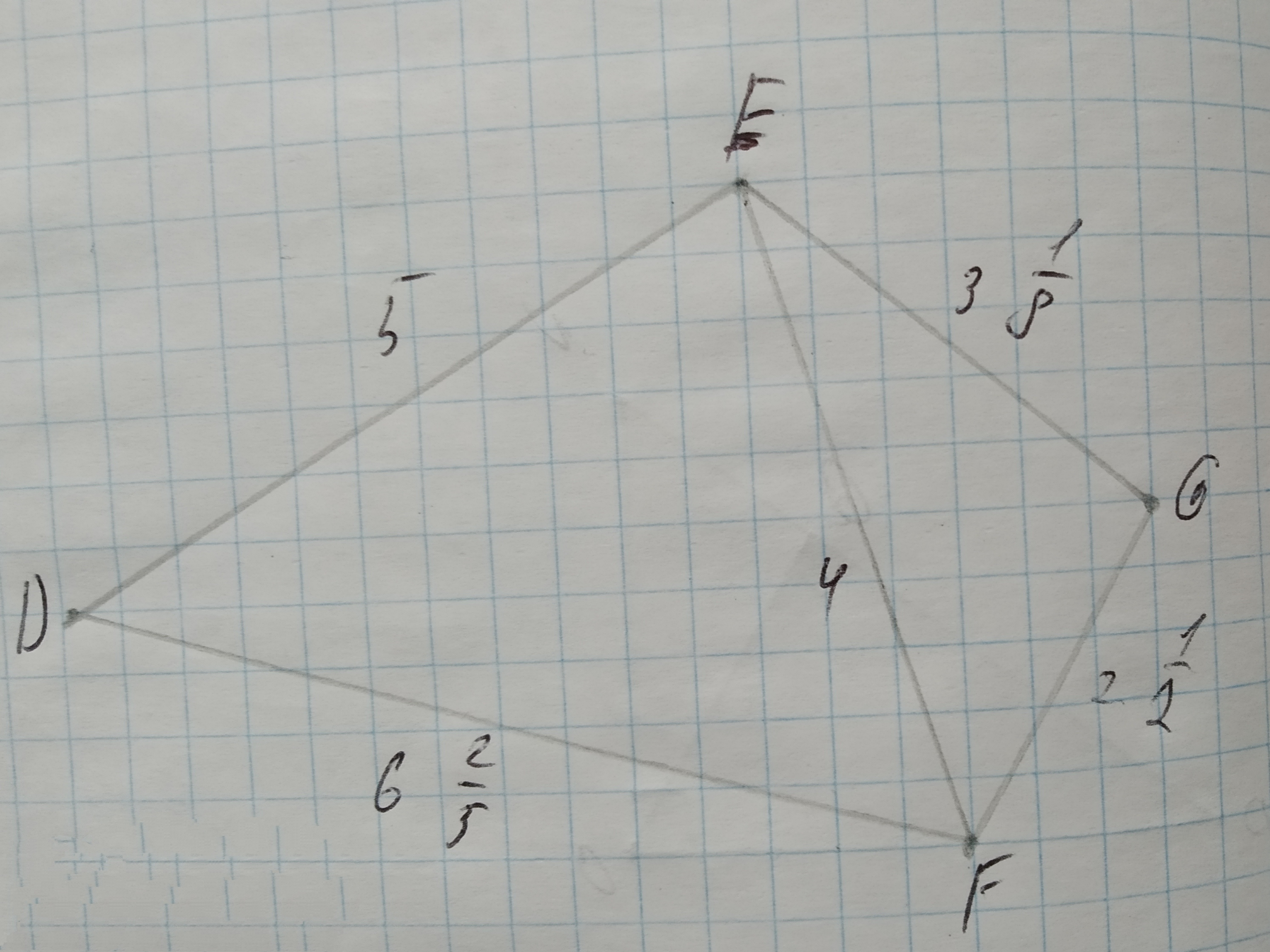The given similarity is $$\displaystyle\triangle{A}{D}{C}\sim\triangle{B}{E}{C}$$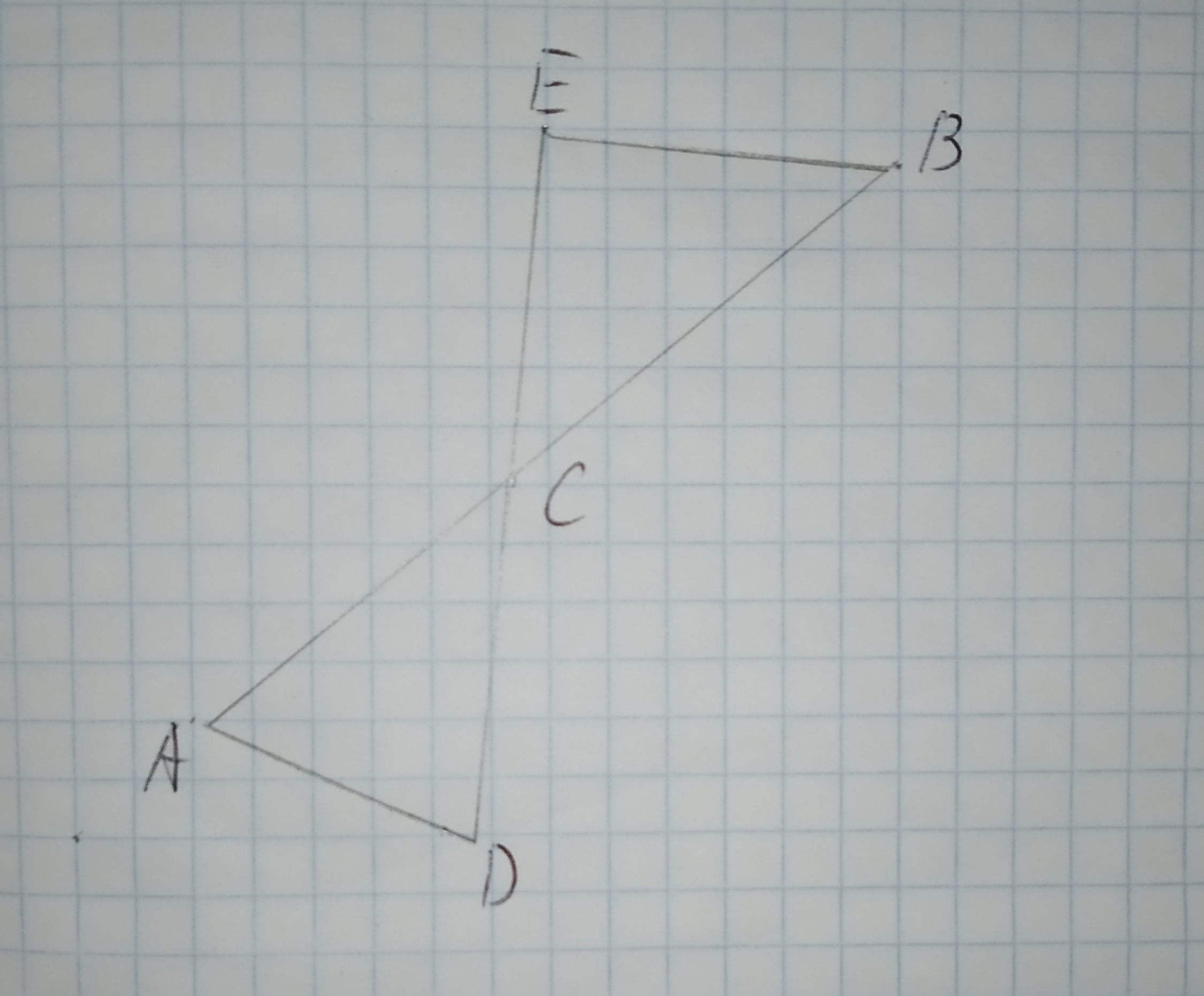A.$$\displaystyle\angle{D}{A}{C}{\quad\text{and}\quad}\angle{E}{C}{B}$$ are congruent.
B.$$\displaystyle\overline{{{A}{C}}}{\quad\text{and}\quad}\overline{{{B}{C}}}$$ are congruent.
C.$$\displaystyle\overline{{{A}{D}}}{\quad\text{and}\quad}\overline{{{E}{B}}}$$ are parallel.
D.$$\displaystyle\angle{C}{E}{B}$$ is a right triangle.